# Recursion Search Recursion the Easy Solution Recursion is

• Slides: 54Recursion, Search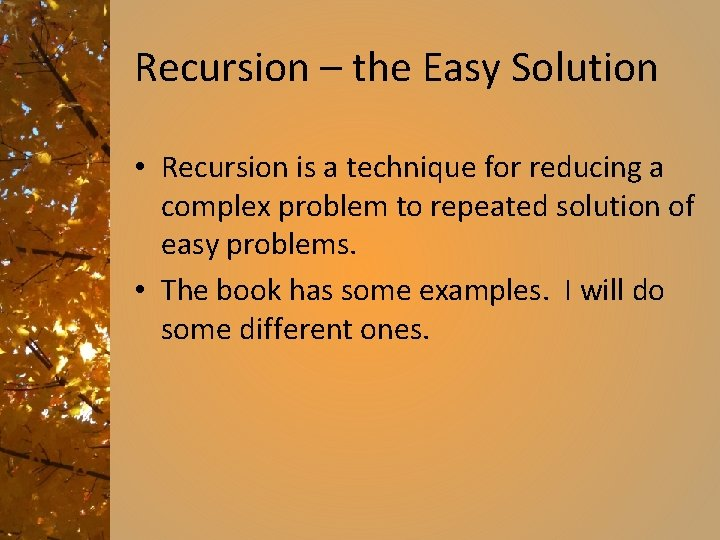Recursion – the Easy Solution • Recursion is a technique for reducing a complex problem to repeated solution of easy problems. • The book has some examples. I will do some different ones.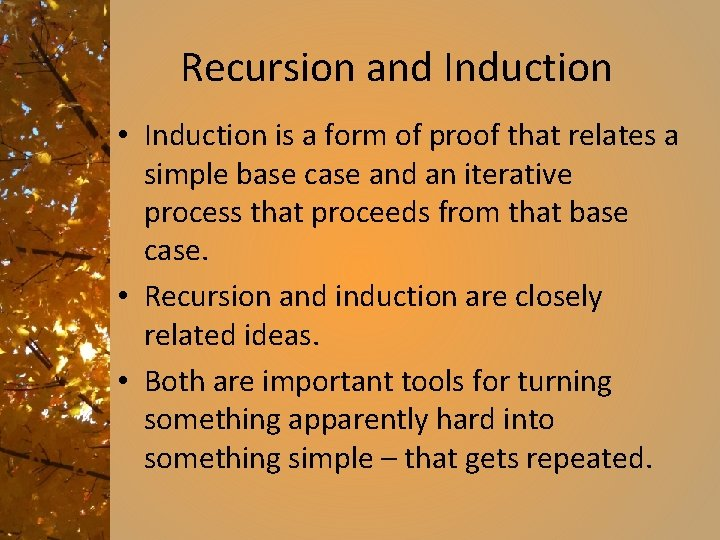Recursion and Induction • Induction is a form of proof that relates a simple base case and an iterative process that proceeds from that base case. • Recursion and induction are closely related ideas. • Both are important tools for turning something apparently hard into something simple – that gets repeated.Towers of Hanoi • My favorite example of recursion. • Puzzle invented by the French mathematician Edouard Lucas in 1883 – Given three pegs, one with a set of disks(8 in the original puzzle) of graduated sizes, – move all the disks to a different peg – never put a larger disk on top of a smaller disk. • See http: //www. mazeworks. com/hanoi/ or http: //www. mathsisfun. com/games/towerofhanoi. html • Solve the puzzle with three disks, then with four disks. • How would you describe the algorithm for solving this puzzle?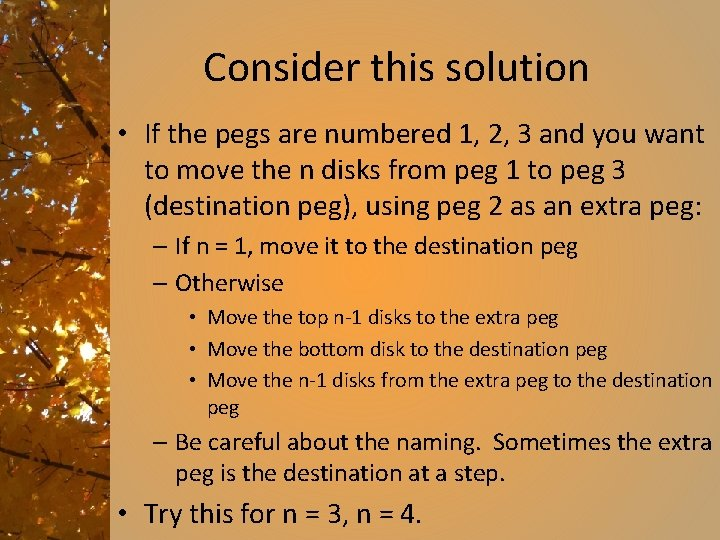Consider this solution • If the pegs are numbered 1, 2, 3 and you want to move the n disks from peg 1 to peg 3 (destination peg), using peg 2 as an extra peg: – If n = 1, move it to the destination peg – Otherwise • Move the top n-1 disks to the extra peg • Move the bottom disk to the destination peg • Move the n-1 disks from the extra peg to the destination peg – Be careful about the naming. Sometimes the extra peg is the destination at a step. • Try this for n = 3, n = 4.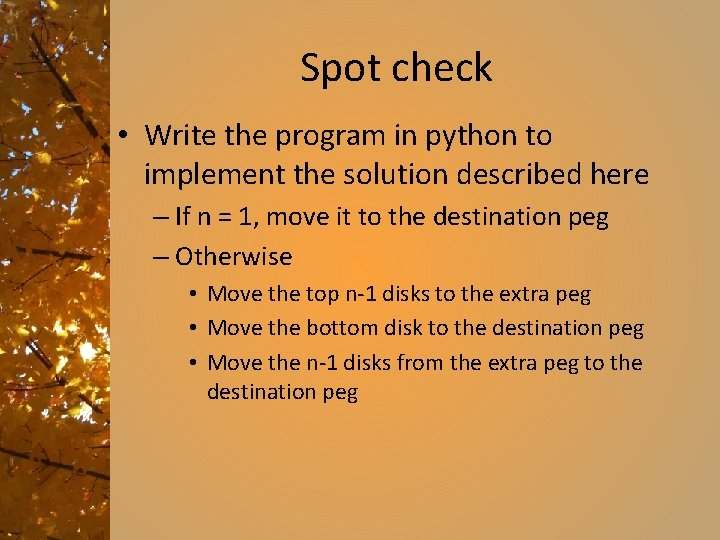Spot check • Write the program in python to implement the solution described here – If n = 1, move it to the destination peg – Otherwise • Move the top n-1 disks to the extra peg • Move the bottom disk to the destination peg • Move the n-1 disks from the extra peg to the destination peg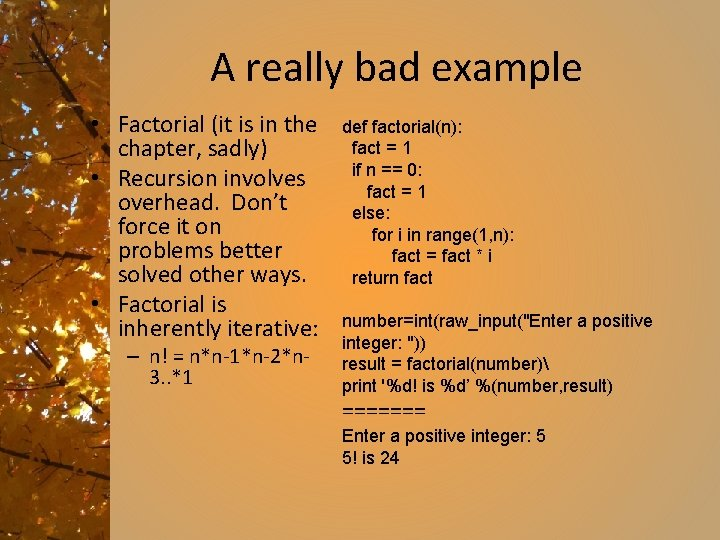A really bad example • Factorial (it is in the chapter, sadly) • Recursion involves overhead. Don’t force it on problems better solved other ways. • Factorial is inherently iterative: – n! = n*n-1*n-2*n 3. . *1 def factorial(n): fact = 1 if n == 0: fact = 1 else: for i in range(1, n): fact = fact * i return fact number=int(raw_input("Enter a positive integer: ")) result = factorial(number) print '%d! is %d’ %(number, result) ======= Enter a positive integer: 5 5! is 24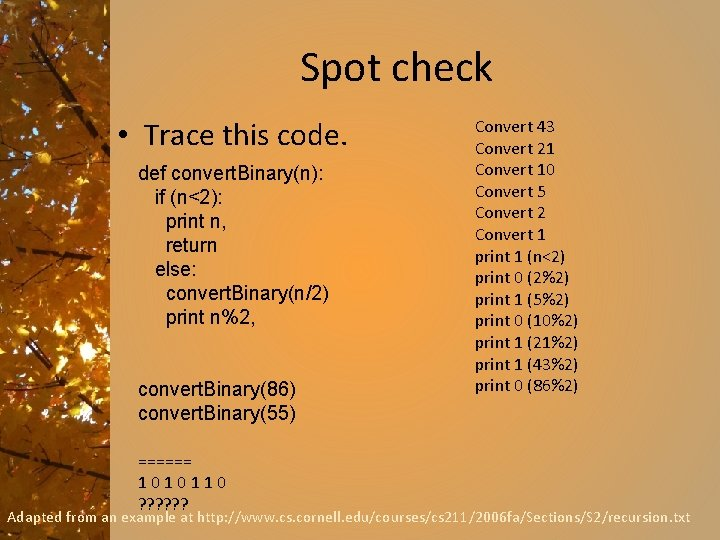Spot check • Trace this code. def convert. Binary(n): if (n<2): print n, return else: convert. Binary(n/2) print n%2, convert. Binary(86) convert. Binary(55) ====== 1010110 ? ? ? Convert 43 Convert 21 Convert 10 Convert 5 Convert 2 Convert 1 print 1 (n<2) print 0 (2%2) print 1 (5%2) print 0 (10%2) print 1 (21%2) print 1 (43%2) print 0 (86%2) Adapted from an example at http: //www. cs. cornell. edu/courses/cs 211/2006 fa/Sections/S 2/recursion. txtFurther examples and explanations • Really good presentation of recursion: – http: //inventwithpython. com/blog/2011/0 8/11/recursion-explained-with-the-flood-fill -algorithm-and-zombies-and-cats/ • Examples of flood fill, more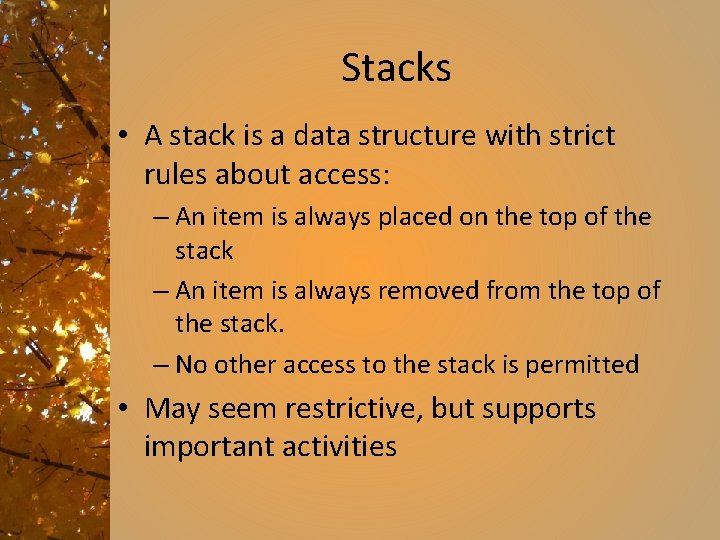Stacks • A stack is a data structure with strict rules about access: – An item is always placed on the top of the stack – An item is always removed from the top of the stack. – No other access to the stack is permitted • May seem restrictive, but supports important activitiesRecursion and Stacks • Recursion works because there are stacks for implementation. • Reexamine the convert. Binary example, for instance: def convert. Binary(n): if (n<2): print n, return else: convert. Binary(n/2) print n%2, convert. Binary(86) On each call, we want to have the most recent results available. A stack is a first in – last out data structure -- FILOImplementing a Stack • A stack is just a collection of items with rules about access. It does not look any different from other collections of items. The thing that makes it a stack is the way it is used. • A list can be used as a stack, if we restrict the operations allowed to append and pop.Push and Pop • The two operations allowed on stacks are called push and pop. Push onto the top of the stack, pop off from the top of the stack. • In python push = append, pop = popApplication of stacks • Arithmetic without parentheses: • Reverse Polish notation – Named for Jan Łukasiewicz, who invented Polish notation – Widely used for computations • Familiar form: infix notation – requires parentheses to determine order of operation: (3+4)*2 • Prefix notation – operator first: * + 3 4 2 • Postfix notation – operator last: 3 4 + 2 *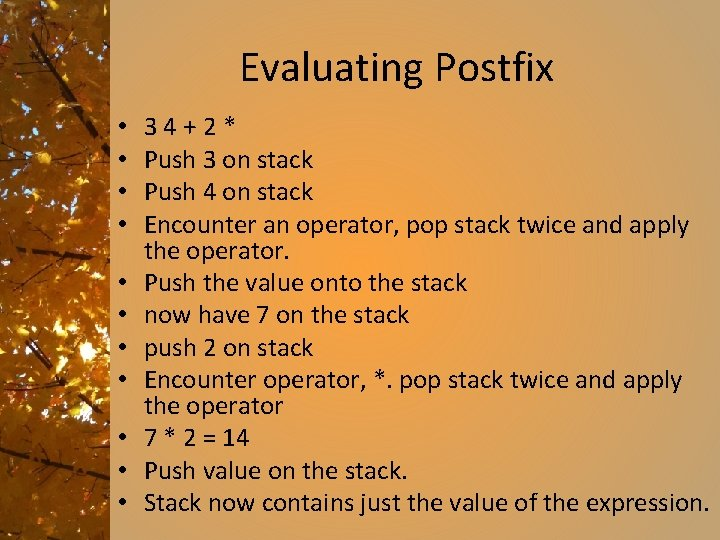Evaluating Postfix • • • 34+2* Push 3 on stack Push 4 on stack Encounter an operator, pop stack twice and apply the operator. Push the value onto the stack now have 7 on the stack push 2 on stack Encounter operator, *. pop stack twice and apply the operator 7 * 2 = 14 Push value on the stack. Stack now contains just the value of the expression.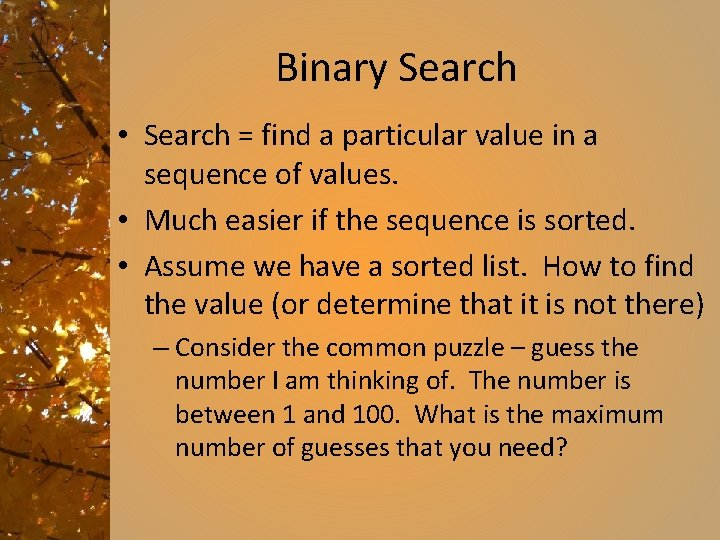Binary Search • Search = find a particular value in a sequence of values. • Much easier if the sequence is sorted. • Assume we have a sorted list. How to find the value (or determine that it is not there) – Consider the common puzzle – guess the number I am thinking of. The number is between 1 and 100. What is the maximum number of guesses that you need?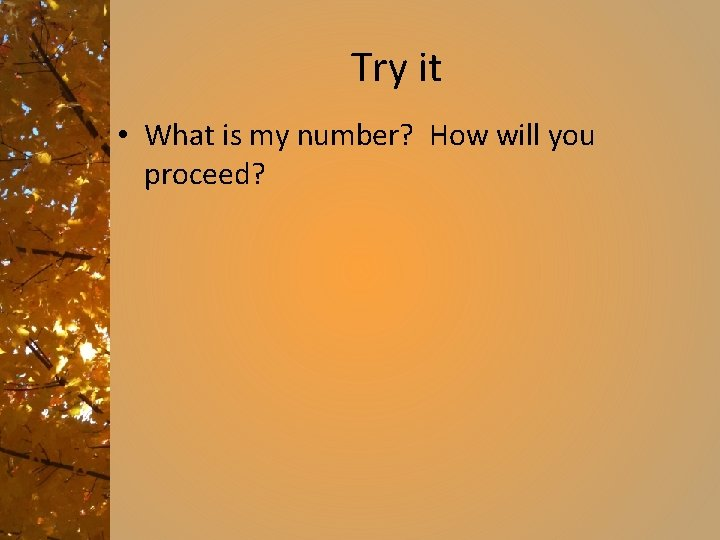Try it • What is my number? How will you proceed?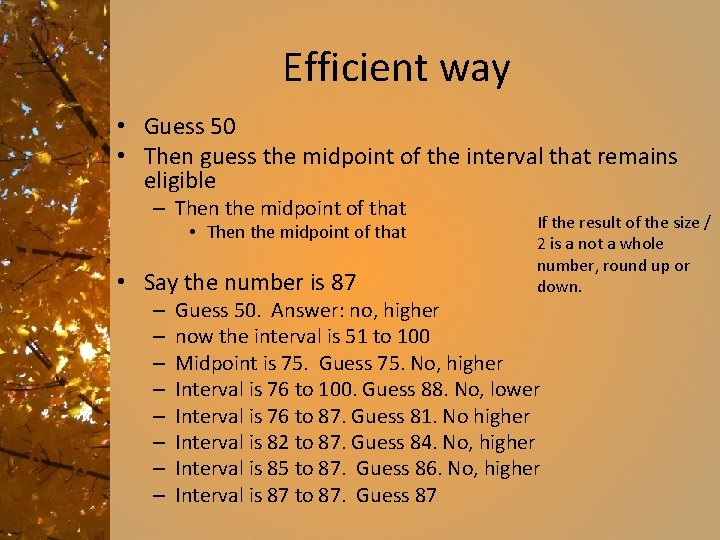Efficient way • Guess 50 • Then guess the midpoint of the interval that remains eligible – Then the midpoint of that • Say the number is 87 – – – – If the result of the size / 2 is a not a whole number, round up or down. Guess 50. Answer: no, higher now the interval is 51 to 100 Midpoint is 75. Guess 75. No, higher Interval is 76 to 100. Guess 88. No, lower Interval is 76 to 87. Guess 81. No higher Interval is 82 to 87. Guess 84. No, higher Interval is 85 to 87. Guess 86. No, higher Interval is 87 to 87. Guess 87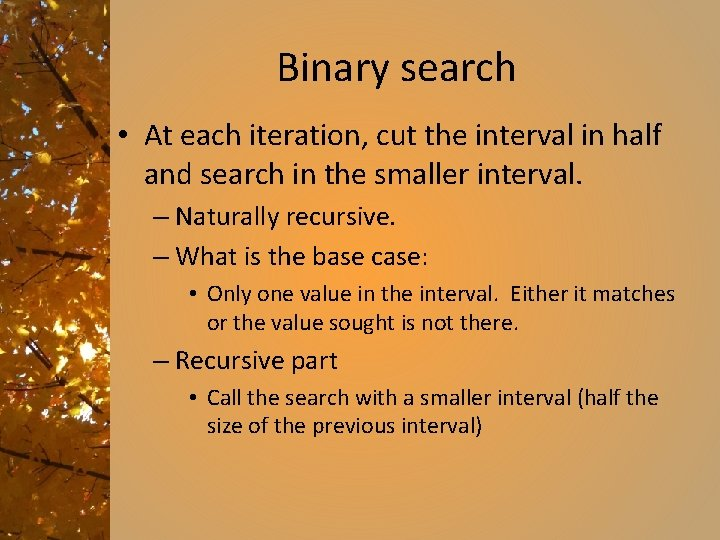Binary search • At each iteration, cut the interval in half and search in the smaller interval. – Naturally recursive. – What is the base case: • Only one value in the interval. Either it matches or the value sought is not there. – Recursive part • Call the search with a smaller interval (half the size of the previous interval)Spot check • Given a list with values: – [“Ashley”, “Emma”, “Heather”, “Madeline”, “Max”, “Sydney”, “Will”] • Answer the question: Is there an entry for “Susan” • Using words, not numbers, but the principle is the same. Show the steps.Spot check again • Work together and work out the basic structure of the binary search. – Don’t worry about getting all the details right, just get the general ideas. • Examine the book version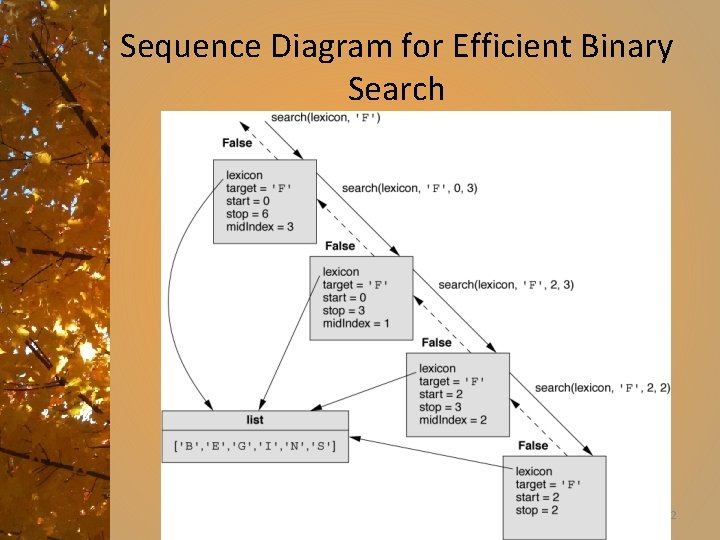Sequence Diagram for Efficient Binary Search 22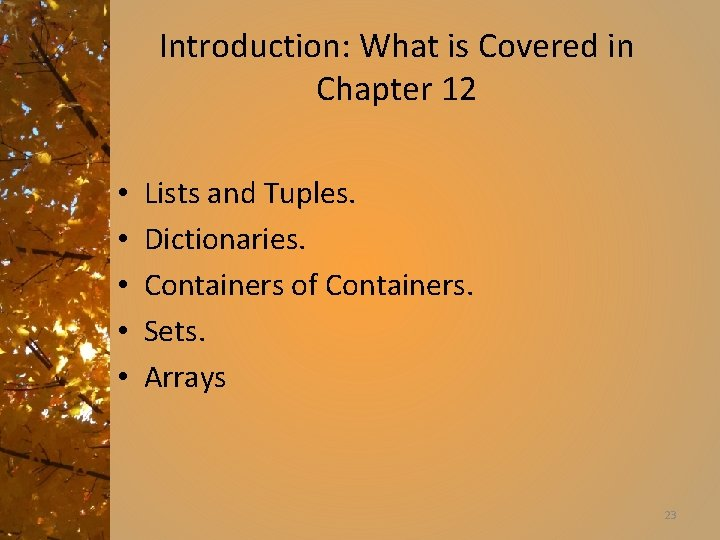Introduction: What is Covered in Chapter 12 • • • Lists and Tuples. Dictionaries. Containers of Containers. Sets. Arrays 23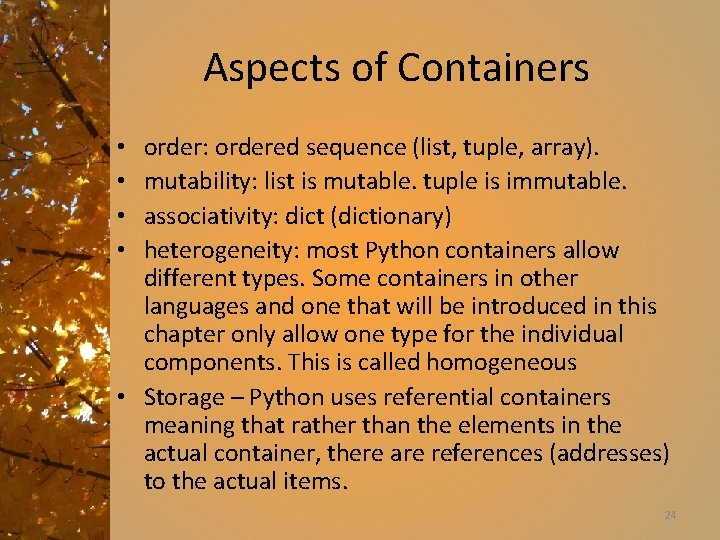Aspects of Containers order: ordered sequence (list, tuple, array). mutability: list is mutable. tuple is immutable. associativity: dict (dictionary) heterogeneity: most Python containers allow different types. Some containers in other languages and one that will be introduced in this chapter only allow one type for the individual components. This is called homogeneous • Storage – Python uses referential containers meaning that rather than the elements in the actual container, there are references (addresses) to the actual items. • • 24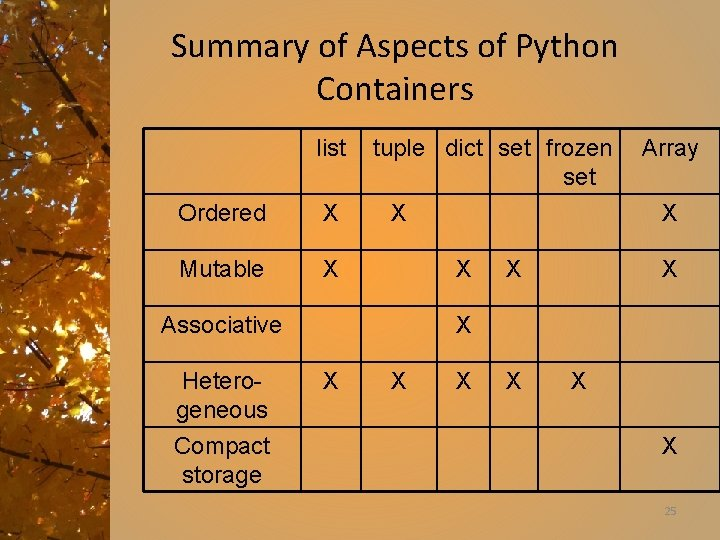Summary of Aspects of Python Containers list Ordered X Mutable X tuple dict set frozen set X Heterogeneous Compact storage X X Associative Array X X X X X 25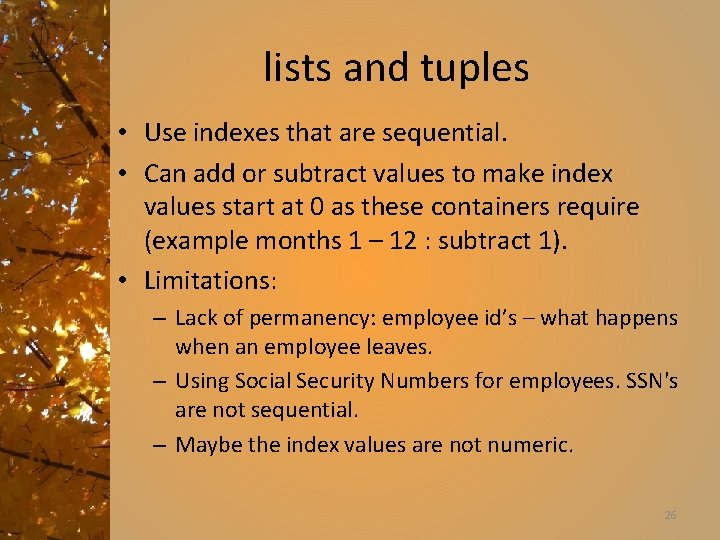lists and tuples • Use indexes that are sequential. • Can add or subtract values to make index values start at 0 as these containers require (example months 1 – 12 : subtract 1). • Limitations: – Lack of permanency: employee id’s – what happens when an employee leaves. – Using Social Security Numbers for employees. SSN's are not sequential. – Maybe the index values are not numeric. 26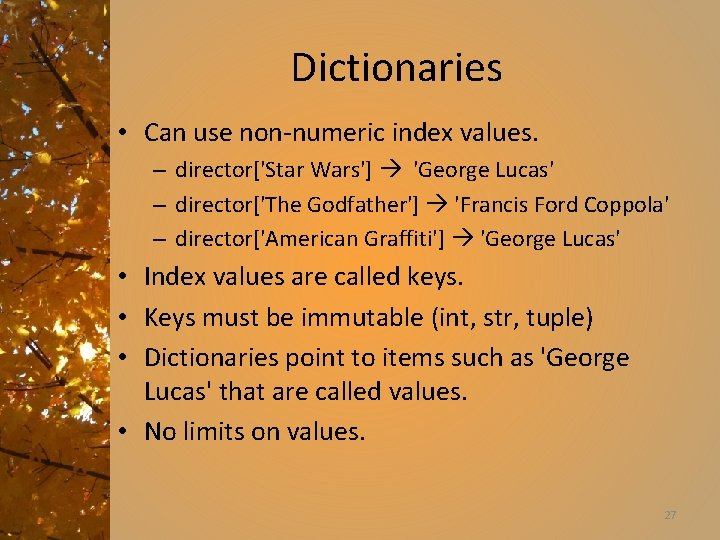Dictionaries • Can use non-numeric index values. – director['Star Wars'] 'George Lucas' – director['The Godfather'] 'Francis Ford Coppola' – director['American Graffiti'] 'George Lucas' • Index values are called keys. • Keys must be immutable (int, str, tuple) • Dictionaries point to items such as 'George Lucas' that are called values. • No limits on values. 27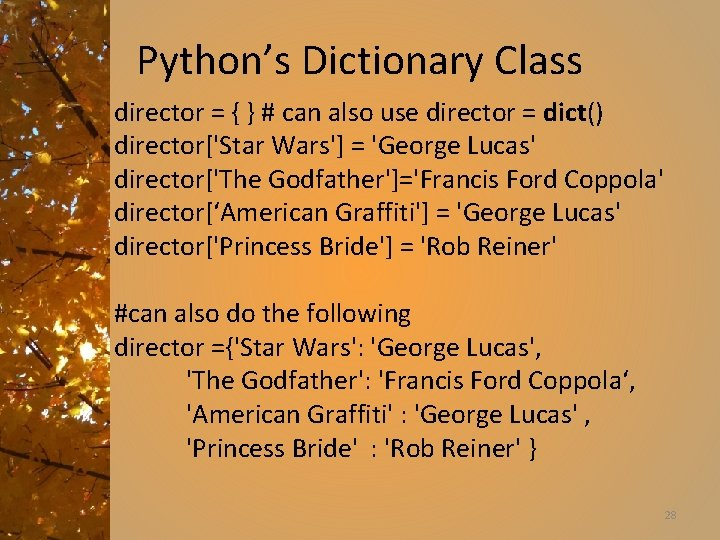Python’s Dictionary Class director = { } # can also use director = dict() director['Star Wars'] = 'George Lucas' director['The Godfather']='Francis Ford Coppola' director[‘American Graffiti'] = 'George Lucas' director['Princess Bride'] = 'Rob Reiner' #can also do the following director ={'Star Wars': 'George Lucas', 'The Godfather': 'Francis Ford Coppola‘, 'American Graffiti' : 'George Lucas' , 'Princess Bride' : 'Rob Reiner' } 28Dictionary Behaviors Syntax Semantics d[k] Returns value at key k, error if k not found. d[k] = value Assigns value to d at key value k. k in d True if k is a member of d else False. len(d) Returns the number of items in d. clear() Make d empty. d. pop(k) Remove key k and return value at k to caller. 29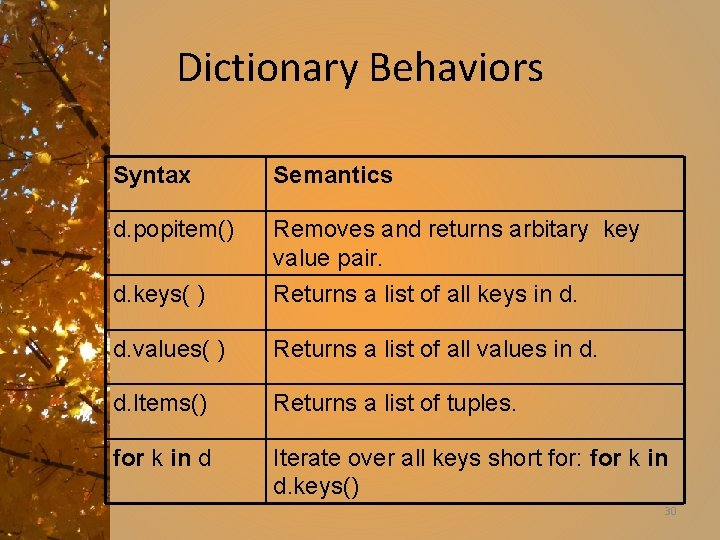Dictionary Behaviors Syntax Semantics d. popitem() Removes and returns arbitary key value pair. d. keys( ) Returns a list of all keys in d. values( ) Returns a list of all values in d. Items() Returns a list of tuples. for k in d Iterate over all keys short for: for k in d. keys() 30Iterating Through Entries of a Dictionary #display a dictionary in sorted order on keys titles = director. keys() titles. sort() for movie in titles: print movie, 'was direct by', director[movie] #can streamline this syntax for movie in sorted(director): print movie, 'was directed by', director[movie] #can iterate over both keys and values for movie, person in director. items(): print movie, 'was directed by', person 31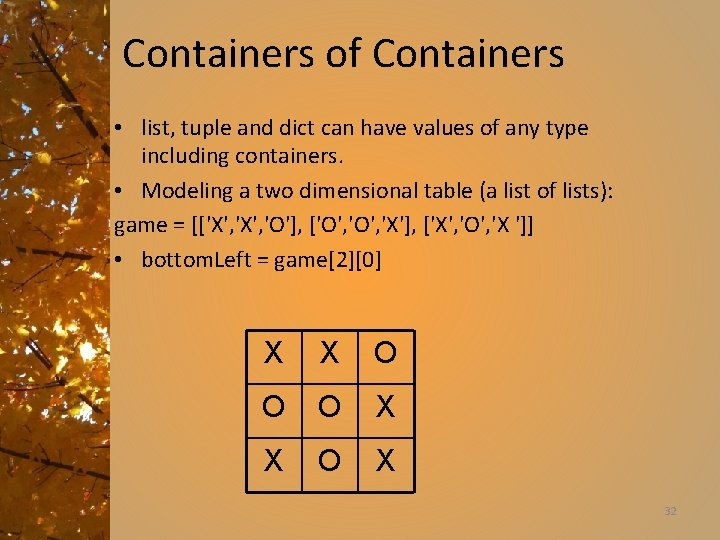Containers of Containers • list, tuple and dict can have values of any type including containers. • Modeling a two dimensional table (a list of lists): game = [['X', 'O'], ['O', 'X'], ['X', 'O', 'X ']] • bottom. Left = game X X O O O X X O X 32Tic-tac-toe Representation 33Modeling Many-to-Many Relationships • Dictionary is a many-to-one relationship. Many keys may map to the same value. • Modeling many-to-many relationship. For example a single movie may have many actors. cast['The Princess Bride '] = ('Cary Elwes', 'Robin Wright Penn', 'Chris Sarandon', 'Mandy Patinkin', 'Andre the Giant', . . . ) 34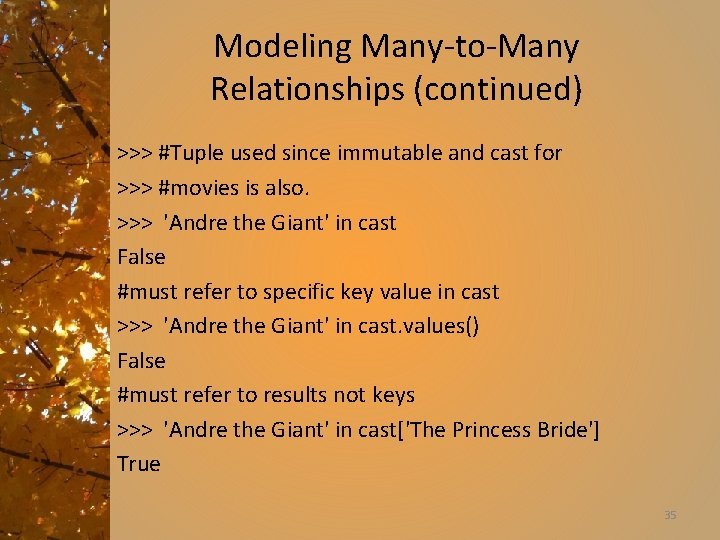Modeling Many-to-Many Relationships (continued) >>> #Tuple used since immutable and cast for >>> #movies is also. >>> 'Andre the Giant' in cast False #must refer to specific key value in cast >>> 'Andre the Giant' in cast. values() False #must refer to results not keys >>> 'Andre the Giant' in cast['The Princess Bride'] True 35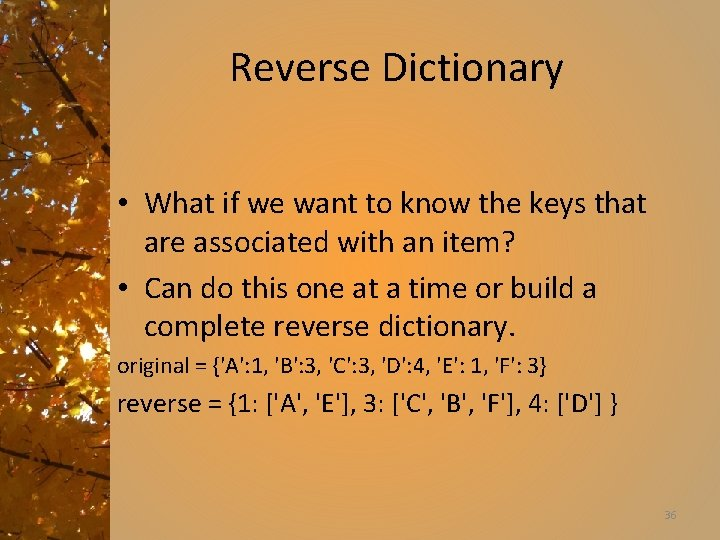Reverse Dictionary • What if we want to know the keys that are associated with an item? • Can do this one at a time or build a complete reverse dictionary. original = {'A': 1, 'B': 3, 'C': 3, 'D': 4, 'E': 1, 'F': 3} reverse = {1: ['A', 'E'], 3: ['C', 'B', 'F'], 4: ['D'] } 36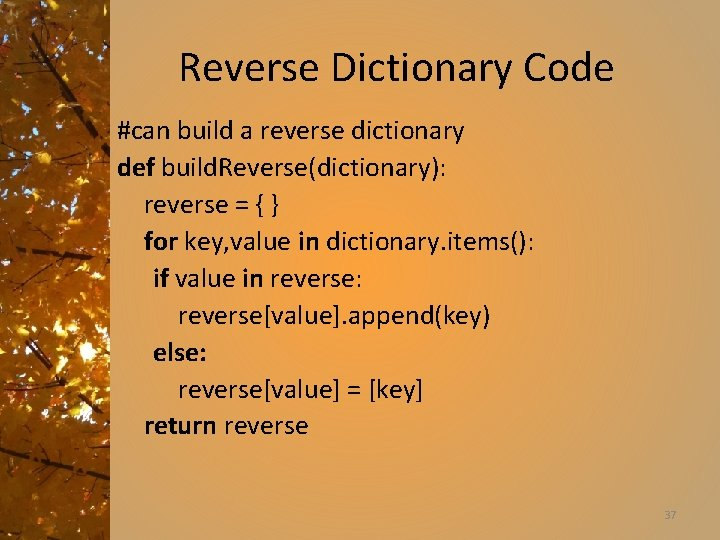Reverse Dictionary Code #can build a reverse dictionary def build. Reverse(dictionary): reverse = { } for key, value in dictionary. items(): if value in reverse: reverse[value]. append(key) else: reverse[value] = [key] return reverse 37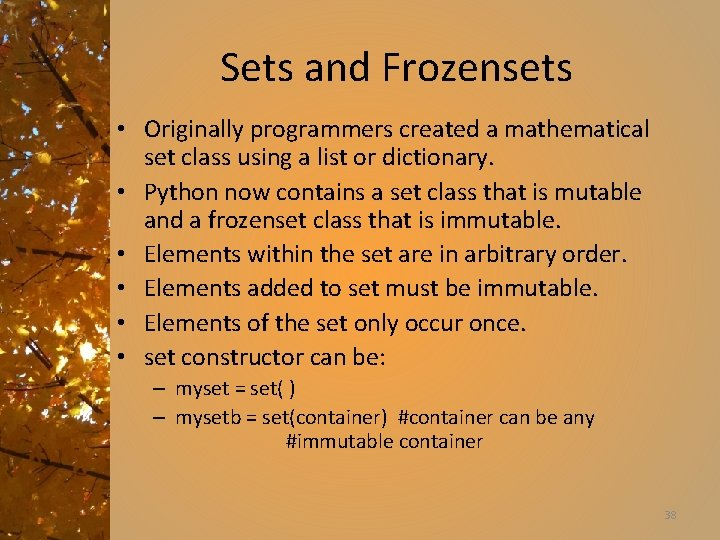Sets and Frozensets • Originally programmers created a mathematical set class using a list or dictionary. • Python now contains a set class that is mutable and a frozenset class that is immutable. • Elements within the set are in arbitrary order. • Elements added to set must be immutable. • Elements of the set only occur once. • set constructor can be: – myset = set( ) – mysetb = set(container) #container can be any #immutable container 38Set Accessor Methods: Standard Container Methods Syntax Semantics len(s) Returns the cardinality of set s. v in s Returns True if v is in set s, else False. v not in s Obviously does opposite of command above. for v in s Iterates over all values in set s in arbitrary order. 39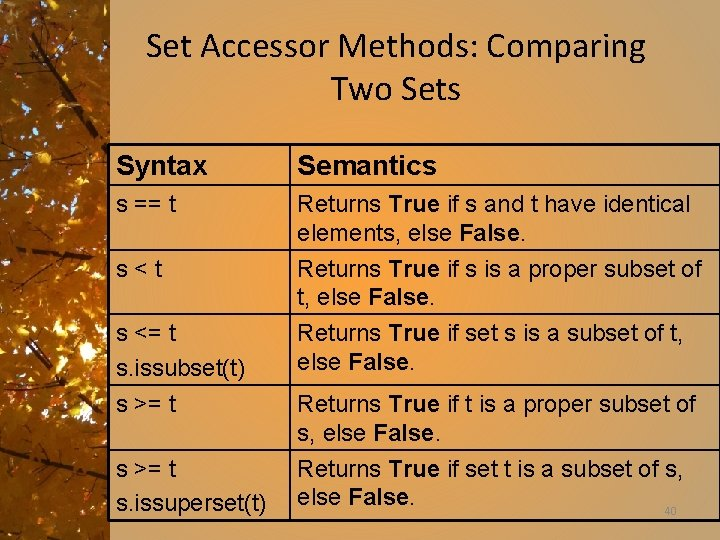Set Accessor Methods: Comparing Two Sets Syntax Semantics s == t Returns True if s and t have identical elements, else False. s<t Returns True if s is a proper subset of t, else False. s <= t s. issubset(t) s >= t Returns True if set s is a subset of t, else False. s >= t s. issuperset(t) Returns True if set t is a subset of s, else False. 40 Returns True if t is a proper subset of s, else False.Set Accessor Methods: Creating a Third Set on Existing Sets Syntax Semantics s|t s. union(t) Returns a new set of elements in either set but with no repeats. s&t s. Intersection(t) Returns a new set of elements that are in both sets s and t. s–t s. difference(t) Returns a new set of elements that are in set s but not in set t. s^t s. symmetric_difference(t) Returns a new set of elements that are in either set s or t but not both. s XOR t. 41Set Mutator Methods Syntax Semantics s. add(v) Adds value v to set s. No effect if v is already in s. discard(v) Removes v from set s. No effect if v is not in s. Removes v from set s if present. Else it raises Key. Error. s. remove(v) s. pop() s. clear() Removes and returns arbitrary value from set s. Removes all entries from the set s. 42Set Mutator Methods (continued) Syntax Semantics s |= t, s. update(t) Alters set s making it the union of sets s and t. s &= t, s. intersection_update(t) Alters set s by making it the intersection of sets s and t. s -= t s. difference_update(t) Alters set s making it s – t. s ^= t s. symmetric_difference_update(t) Alters set s making it the XOR of s and t. 43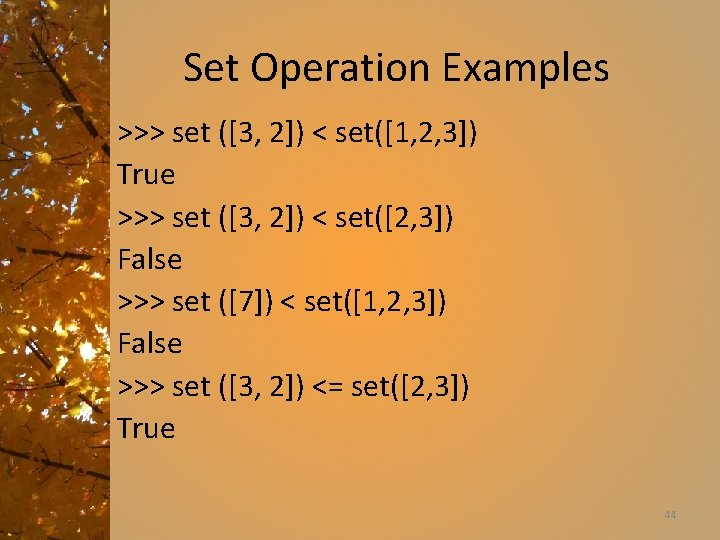Set Operation Examples >>> set ([3, 2]) < set([1, 2, 3]) True >>> set ([3, 2]) < set([2, 3]) False >>> set () < set([1, 2, 3]) False >>> set ([3, 2]) <= set([2, 3]) True 44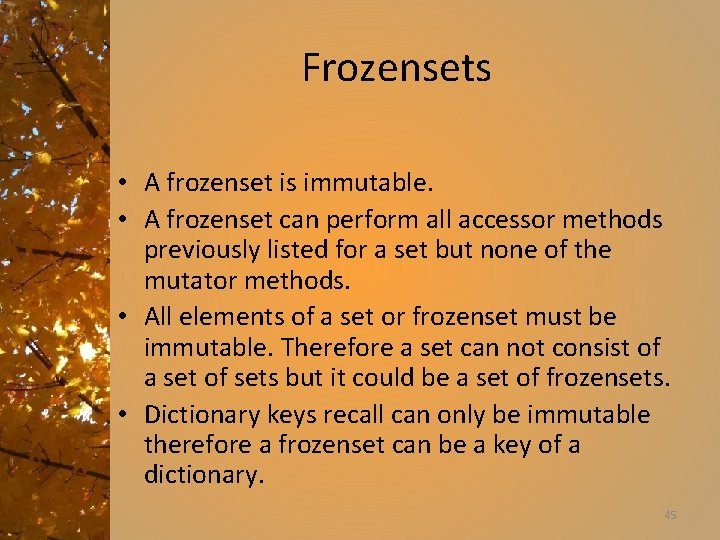Frozensets • A frozenset is immutable. • A frozenset can perform all accessor methods previously listed for a set but none of the mutator methods. • All elements of a set or frozenset must be immutable. Therefore a set can not consist of a set of sets but it could be a set of frozensets. • Dictionary keys recall can only be immutable therefore a frozenset can be a key of a dictionary. 45Sets and Frozensets • Two sets operated on results in a set. • Two frozensets operated on results in a frozenset. • A set and a frozenset results in the type that occurs first in the operation. 46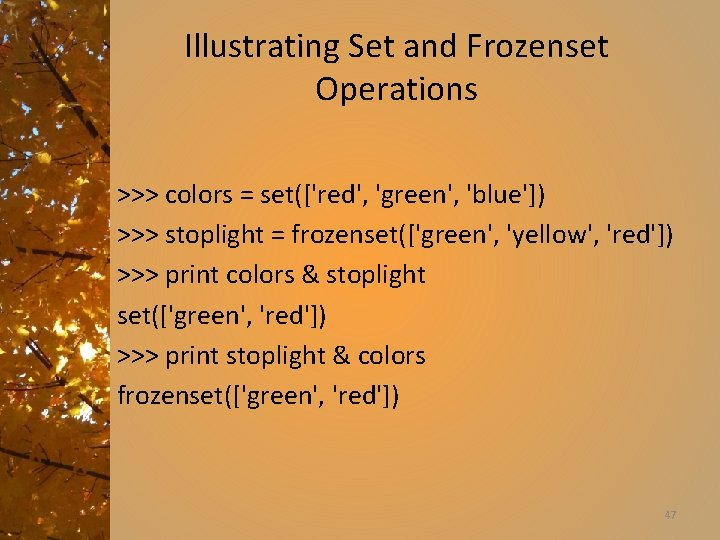Illustrating Set and Frozenset Operations >>> colors = set(['red', 'green', 'blue']) >>> stoplight = frozenset(['green', 'yellow', 'red']) >>> print colors & stoplight set(['green', 'red']) >>> print stoplight & colors frozenset(['green', 'red']) 47Lists Versus Arrays • A list consists of a list of references to items. • Advantage is that a list can consist of different types. This is called a heterogeneous type. • Disadvantage is slightly slower access since it requires two accesses to retrieve an item (once to get the reference and once to retrieve the value from the reference). 48Lists Versus Arrays (continued) • The array type consists of the actual items. • Homogenous data structure. • Slightly faster access than a list since only one access is required to retrieve a value. 49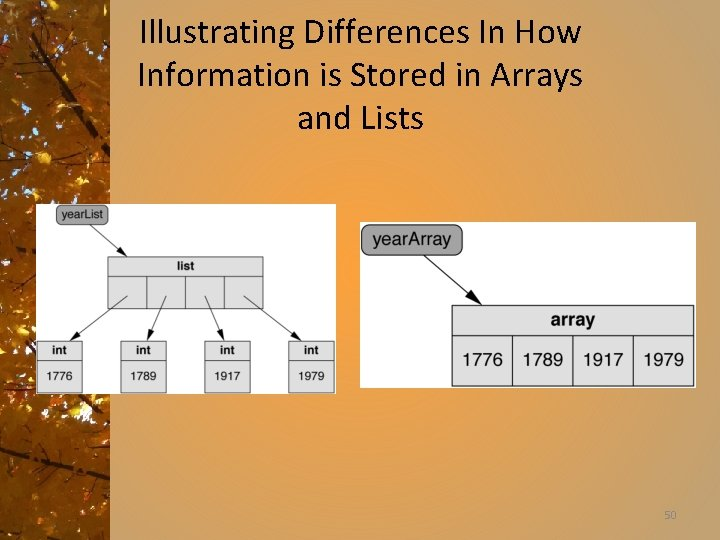Illustrating Differences In How Information is Stored in Arrays and Lists 50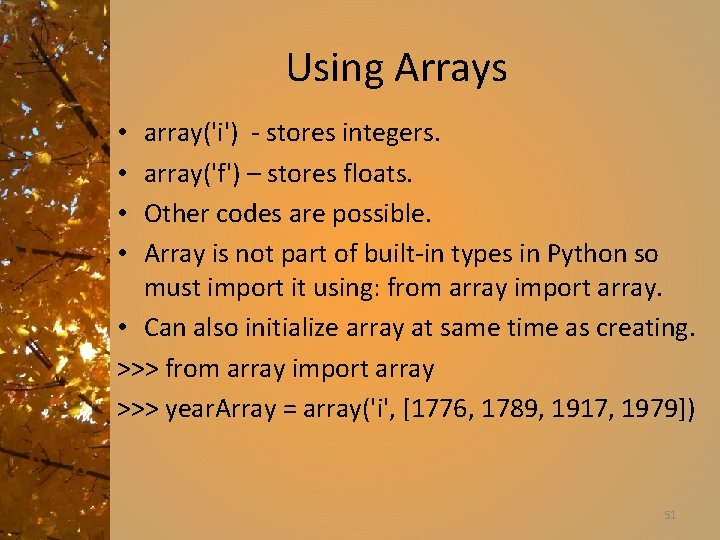Using Arrays array('i') - stores integers. array('f') – stores floats. Other codes are possible. Array is not part of built-in types in Python so must import it using: from array import array. • Can also initialize array at same time as creating. >>> from array import array >>> year. Array = array('i', [1776, 1789, 1917, 1979]) • • 51Array content types from: http: //docs. python. org/library/array. html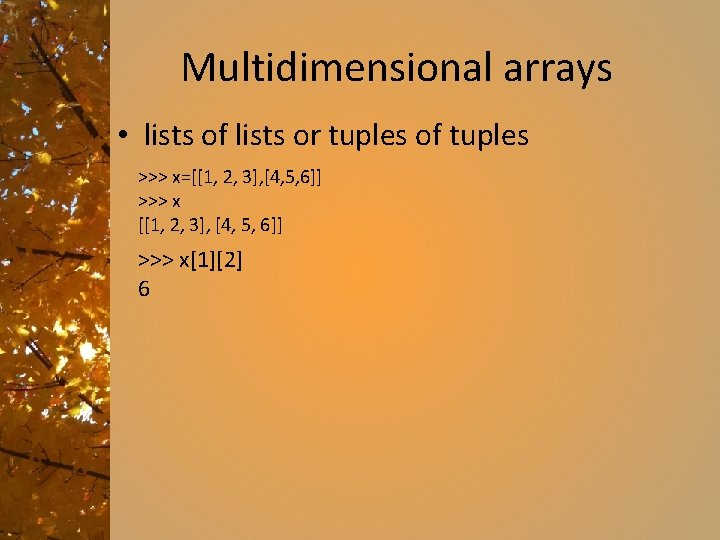Multidimensional arrays • lists of lists or tuples of tuples >>> x=[[1, 2, 3], [4, 5, 6]] >>> x 6Exercise and Project • Exercise 11. 38 – Due two weeks – 15 November